Courses

# Greedy Computer Science Engineering (CSE) Notes | EduRev

## Computer Science Engineering (CSE) : Greedy Computer Science Engineering (CSE) Notes | EduRev

The document Greedy Computer Science Engineering (CSE) Notes | EduRev is a part of the Computer Science Engineering (CSE) Course GATE Computer Science Engineering(CSE) 2022 Mock Test Series.
All you need of Computer Science Engineering (CSE) at this link: Computer Science Engineering (CSE)

## Greedy Algorithms | (Activity Selection Problem)

Greedy is an algorithmic paradigm that builds up a solution piece by piece, always choosing the next piece that offers the most obvious and immediate benefit. Greedy algorithms are used for optimization problems. An optimization problem can be solved using Greedy if the problem has the following property: At every step, we can make a choice that looks best at the moment, and we get the optimal solution of the complete problem.
If a Greedy Algorithm can solve a problem, then it generally becomes the best method to solve that problem as the Greedy algorithms are in general more efficient than other techniques like Dynamic Programming. But Greedy algorithms cannot always be applied. For example, Fractional Knapsack problem can be solved using Greedy, but  0-1 Knapsack cannot be solved using Greedy.

Following are some standard algorithms that are Greedy algorithms.
1)  Kruskal`s Minuimum Spanning tree (MST): In Kruskal’s algorithm, we create a MST by picking edges one by one. The Greedy Choice is to pick the smallest weight edge that doesn’t cause a cycle in the MST constructed so far.
2)  Prim`s Minimum Spanning Tree: In Prim’s algorithm also, we create a MST by picking edges one by one. We maintain two sets: set of the vertices already included in MST and the set of the vertices not yet included. The Greedy Choice is to pick the smallest weight edge that connects the two sets.
3)  Dijkstra`s Shortest Path: The Dijkstra’s algorithm is very similar to Prim’s algorithm. The shortest path tree is built up, edge by edge. We maintain two sets: set of the vertices already included in the tree and the set of the vertices not yet included. The Greedy Choice is to pick the edge that connects the two sets and is on the smallest weight path from source to the set that contains not yet included vertices.
4)  Huffman Coding: Huffman Coding is a loss-less compression technique. It assigns variable length bit codes to different characters. The Greedy Choice is to assign least bit length code to the most frequent character.

The greedy algorithms are sometimes also used to get an approximation for Hard optimization problems. For example,  Traveling Salesman Problem is a NP Hard problem. A Greedy choice for this problem is to pick the nearest unvisited city from the current city at every step. This solutions doesn’t always produce the best optimal solution, but can be used to get an approximate optimal solution.

Let us consider the  Actvity Selection problem as our first example of Greedy algorithms. Following is the problem statement.
You are given n activities with their start and finish times. Select the maximum number of activities that can be performed by a single person, assuming that a person can only work on a single activity at a time.
Example:

Example 1 : Consider the following 3 activities sorted by
by finish time.
start[]  =  {10, 12, 20};
finish[] =  {20, 25, 30};
A person can perform at most two activities. The
maximum set of activities that can be executed
is {0, 2} [ These are indexes in start[] and
finish[] ]

Example 2 : Consider the following 6 activities
sorted by by finish time.
start[]  =  {1, 3, 0, 5, 8, 5};
finish[] =  {2, 4, 6, 7, 9, 9};
A person can perform at most four activities. The
maximum set of activities that can be executed
is {0, 1, 3, 4} [ These are indexes in start[] and
finish[] ]

The greedy choice is to always pick the next activity whose finish time is least among the remaining activities and the start time is more than or equal to the finish time of previously selected activity. We can sort the activities according to their finishing time so that we always consider the next activity as minimum finishing time activity.

1) Sort the activities according to their finishing time
2) Select the first activity from the sorted array and print it.
3) Do following for remaining activities in the sorted array.
…….a) If the start time of this activity is greater than or equal to the finish time of previously selected activity then select this activity and print it.

In the following C implementation, it is assumed that the activities are already sorted according to their finish time.

// C++ program for activity selection problem.
// The following implementation assumes that the activities
// are already sorted according to their finish time
#include<stdio.h>

// Prints a maximum set of activities that can be done by a single
// person, one at a time.
//  n   -->  Total number of activities
//  s[] -->  An array that contains start time of all activities
//  f[] -->  An array that contains finish time of all activities
void printMaxActivities(int s[], int f[], int n)
{
int i, j;

printf ("Following activities are selected ");

// The first activity always gets selected
i = 0;
printf("%d ", i);

// Consider rest of the activities
for (j = 1; j < n; j++)
{
// If this activity has start time greater than or
// equal to the finish time of previously selected
// activity, then select it
if (s[j] >= f[i])
{
printf ("%d ", j);
i = j;
}
}
}

// driver program to test above function
int main()
{
int s[] =  {1, 3, 0, 5, 8, 5};
int f[] =  {2, 4, 6, 7, 9, 9};
int n = sizeof(s)/sizeof(s);
printMaxActivities(s, f, n);
return 0;
}

Output:
Following activities are selected
0 1 3 4

How does Greedy Choice work for Activities sorted according to finish time?

Let the give set of activities be S = {1, 2, 3, ..n} and activities be sorted by finish time. The greedy choice is to always pick activity 1. How come the activity 1 always provides one of the optimal solutions. We can prove it by showing that if there is another solution B with first activity other than 1, then there is also a solution A of same size with activity 1 as first activity. Let the first activity selected by B be k, then there always exist A = {B – {k}} U {1}.(Note that the activities in B are independent and k has smallest finishing time among all. Since k is not 1, finish(k) >= finish(1)).

How to implement when given activities are not sorted?

We create a structure/class for activities. We sort all activities by finish time. Once we have activities sorted, we apply same above algorithm.

// C++ program for activity selection problem
// when input activities may not be sorted.
#include <bits/stdc++.h>
using namespace std;

// A job has start time, finish time and profit.
struct Activitiy
{
int start, finish;
};

// A utility function that is used for sorting
// activities according to finish time
bool activityCompare(Activitiy s1, Activitiy s2)
{
return (s1.finish < s2.finish);
}

// Returns count of maximum set of activities that can
// be done by a single person, one at a time.
void printMaxActivities(Activitiy arr[], int n)
{
// Sort jobs according to finish time
sort(arr, arr+n, activityCompare);

cout << "Following activities are selected ";

// The first activity always gets selected
int i = 0;
cout << "(" << arr[i].start << ", " << arr[i].finish << "), ";

// Consider rest of the activities
for (int j = 1; j < n; j++)
{
// If this activity has start time greater than or
// equal to the finish time of previously selected
// activity, then select it
if (arr[j].start >= arr[i].finish)
{
cout << "(" << arr[j].start << ", "
<< arr[j].finish << "), ";
i = j;
}
}
}

// Driver program
int main()
{
Activitiy arr[] = {{5, 9}, {1, 2}, {3, 4}, {0, 6},
{5, 7}, {8, 9}};
int n = sizeof(arr)/sizeof(arr);
printMaxActivities(arr, n);
return 0;
}

Output:

Following activities are selected
(1, 2), (3, 4), (5, 7), (8, 9),

Time Complexity : It takes O(n log n) time if input activities may not be sorted. It takes O(n) time when it is given that input activities are always sorted.

## Greedy Algorithms | (Kruskal’s Minimum Spanning Tree Algorithm)

What is Minimum Spanning Tree?

Given a connected and undirected graph, a spanning tree of that graph is a subgraph that is a tree and connects all the vertices together. A single graph can have many different spanning trees. A minimum spanning tree (MST) or minimum weight spanning tree for a weighted, connected and undirected graph is a spanning tree with weight less than or equal to the weight of every other spanning tree. The weight of a spanning tree is the sum of weights given to each edge of the spanning tree.

How many edges does a minimum spanning tree has?
A minimum spanning tree has (V – 1) edges where V is the number of vertices in the given graph.

What are the applications of Minimum Spanning Tree?
Below are the steps for finding MST using Kruskal’s algorithm

1. Sort all the edges in non-decreasing order of their weight.

2. Pick the smallest edge. Check if it forms a cycle with the spanning tree
formed so far. If cycle is not formed, include this edge. Else, discard it.

3. Repeat step#2 until there are (V-1) edges in the spanning tree.

The step#2 uses  Union -Find algorithm to detect cycle. So we recommend to read following post as a prerequisite.

The algorithm is a Greedy Algorithm. The Greedy Choice is to pick the smallest weight edge that does not cause a cycle in the MST constructed so far. Let us understand it with an example: Consider the below input graph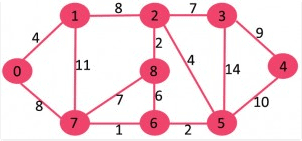The graph contains 9 vertices and 14 edges. So, the minimum spanning tree formed will be having (9 – 1) = 8 edges.

After sorting:
Weight   Src    Dest
1         7      6
2         8      2
2         6      5
4         0      1
4         2      5
6         8      6
7         2      3
7         7      8
8         0      7
8         1      2
9         3      4
10        5      4
11        1      7
14        3      5

Now pick all edges one by one from sorted list of edges
1. Pick edge 7-6: No cycle is formed, include it.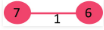2. Pick edge 8-2: No cycle is formed, include it.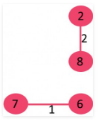3. Pick edge 6-5: No cycle is formed, include it.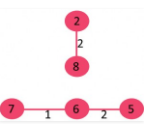4. Pick edge 0-1: No cycle is formed, include it.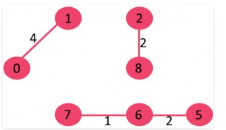5. Pick edge 2-5: No cycle is formed, include it.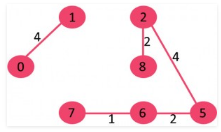6. Pick edge 8-6: Since including this edge results in cycle, discard it.

7. Pick edge 2-3: No cycle is formed, include it.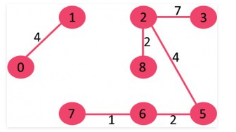8. Pick edge 7-8: Since including this edge results in cycle, discard it.

9. Pick edge 0-7: No cycle is formed, include it.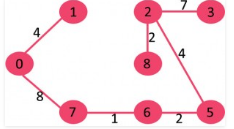10. Pick edge 1-2: Since including this edge results in cycle, discard it.

11. Pick edge 3-4: No cycle is formed, include it.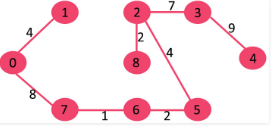Since the number of edges included equals (V – 1), the algorithm stops here.

## Greedy Algorithms | (Huffman Coding)

Huffman coding is a lossless data compression algorithm. The idea is to assign variable-legth codes to input characters, lengths of the assigned codes are based on the frequencies of corresponding characters. The most frequent character gets the smallest code and the least frequent character gets the largest code.
The variable-length codes assigned to input characters are  Prefix Codes, means the codes (bit sequences) are assigned in such a way that the code assigned to one character is not prefix of code assigned to any other character. This is how Huffman Coding makes sure that there is no ambiguity when decoding the generated bit stream.
Let us understand prefix codes with a counter example. Let there be four characters a, b, c and d, and their corresponding variable length codes be 00, 01, 0 and 1. This coding leads to ambiguity because code assigned to c is prefix of codes assigned to a and b. If the compressed bit stream is 0001, the de-compressed output may be “cccd” or “ccb” or “acd” or “ab”.

There are mainly two major parts in Huffman Coding
1) Build a Huffman Tree from input characters.
2) Traverse the Huffman Tree and assign codes to characters.

Steps to build Huffman Tree

Input is array of unique characters along with their frequency of occurrences and output is Huffman Tree.

1. Create a leaf node for each unique character and build a min heap of all leaf nodes (Min Heap is used as a priority queue. The value of frequency field is used to compare two nodes in min heap. Initially, the least frequent character is at root)

2. Extract two nodes with the minimum frequency from the min heap.

3. Create a new internal node with frequency equal to the sum of the two nodes frequencies. Make the first extracted node as its left child and the other extracted node as its right child. Add this node to the min heap.

4. Repeat steps#2 and #3 until the heap contains only one node. The remaining node is the root node and the tree is complete.

Let us understand the algorithm with an example

character   Frequency
a                5
b                9
c               12
d               13
e               16
f                 45

Step 1. Build a min heap that contains 6 nodes where each node represents root of a tree with single node.

Step 2 Extract two minimum frequency nodes from min heap. Add a new internal node with frequency 5 + 9 = 14.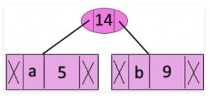Now min heap contains 5 nodes where 4 nodes are roots of trees with single element each, and one heap node is root of tree with 3 elements

character           Frequency
c                        12
d                        13
Internal Node         14
e                        16
f                         45

Step 3: Extract two minimum frequency nodes from heap. Add a new internal node with frequency 12 + 13 = 25Now min heap contains 4 nodes where 2 nodes are roots of trees with single element each, and two heap nodes are root of tree with more than one nodes.

character           Frequency
Internal Node          14
e                         16
Internal Node          25
f                           45

Step 4: Extract two minimum frequency nodes. Add a new internal node with frequency 14 + 16 = 30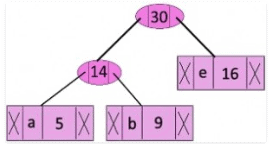Now min heap contains 3 nodes.

character          Frequency
Internal Node         25
Internal Node         30
f                          45

Step 5: Extract two minimum frequency nodes. Add a new internal node with frequency 25 + 30 = 55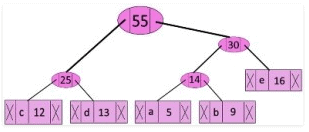Now min heap contains 2 nodes.

character     Frequency
f                    45
Internal Node    55

Step 6: Extract two minimum frequency nodes. Add a new internal node with frequency 45 + 55 = 100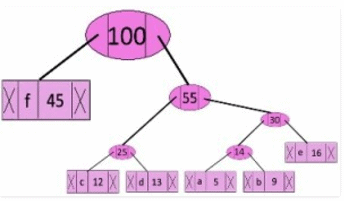Now min heap contains only one node.

character      Frequency
Internal Node    100

Since the heap contains only one node, the algorithm stops here.

Steps to print codes from Huffman Tree:

Traverse the tree formed starting from the root. Maintain an auxiliary array. While moving to the left child, write 0 to the array. While moving to the right child, write 1 to the array. Print the array when a leaf node is encountered.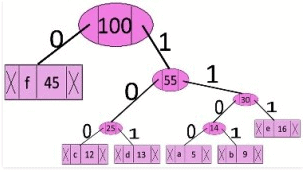The codes are as follows:

character   code-word
f          0
c          100
d          101
a          1100
b          1101
e          111

// C program for Huffman Coding
#include <stdio.h>
#include <stdlib.h>

// This constant can be avoided by explicitly calculating height of Huffman Tree
#define MAX_TREE_HT 100

// A Huffman tree node
struct MinHeapNode
{
char data;  // One of the input characters
unsigned freq;  // Frequency of the character
struct MinHeapNode *left, *right; // Left and right child of this node
};

// A Min Heap:  Collection of min heap (or Hufmman tree) nodes
struct MinHeap
{
unsigned size;    // Current size of min heap
unsigned capacity;   // capacity of min heap
struct MinHeapNode **array;  // Attay of minheap node pointers
};

// A utility function allocate a new min heap node with given character
// and frequency of the character
struct MinHeapNode* newNode(char data, unsigned freq)
{
struct MinHeapNode* temp =
(struct MinHeapNode*) malloc(sizeof(struct MinHeapNode));
temp->left = temp->right = NULL;
temp->data = data;
temp->freq = freq;
return temp;
}

// A utility function to create a min heap of given capacity
struct MinHeap* createMinHeap(unsigned capacity)
{
struct MinHeap* minHeap =
(struct MinHeap*) malloc(sizeof(struct MinHeap));
minHeap->size = 0;  // current size is 0
minHeap->capacity = capacity;
minHeap->array =
(struct MinHeapNode**)malloc(minHeap->capacity * sizeof(struct MinHeapNode*));
return minHeap;
}

// A utility function to swap two min heap nodes
void swapMinHeapNode(struct MinHeapNode** a, struct MinHeapNode** b)
{
struct MinHeapNode* t = *a;
*a = *b;
*b = t;
}

// The standard minHeapify function.
void minHeapify(struct MinHeap* minHeap, int idx)
{
int smallest = idx;
int left = 2 * idx + 1;
int right = 2 * idx + 2;

if (left < minHeap->size &&
minHeap->array[left]->freq < minHeap->array[smallest]->freq)
smallest = left;

if (right < minHeap->size &&
minHeap->array[right]->freq < minHeap->array[smallest]->freq)
smallest = right;

if (smallest != idx)
{
swapMinHeapNode(&minHeap->array[smallest], &minHeap->array[idx]);
minHeapify(minHeap, smallest);
}
}

// A utility function to check if size of heap is 1 or not
int isSizeOne(struct MinHeap* minHeap)
{
return (minHeap->size == 1);
}

// A standard function to extract minimum value node from heap
struct MinHeapNode* extractMin(struct MinHeap* minHeap)
{
struct MinHeapNode* temp = minHeap->array;
minHeap->array = minHeap->array[minHeap->size - 1];
--minHeap->size;
minHeapify(minHeap, 0);
return temp;
}

// A utility function to insert a new node to Min Heap
void insertMinHeap(struct MinHeap* minHeap, struct MinHeapNode* minHeapNode)
{
++minHeap->size;
int i = minHeap->size - 1;
while (i && minHeapNode->freq < minHeap->array[(i - 1)/2]->freq)
{
minHeap->array[i] = minHeap->array[(i - 1)/2];
i = (i - 1)/2;
}
minHeap->array[i] = minHeapNode;
}

// A standard funvtion to build min heap
void buildMinHeap(struct MinHeap* minHeap)
{
int n = minHeap->size - 1;
int i;
for (i = (n - 1) / 2; i >= 0; --i)
minHeapify(minHeap, i);
}

// A utility function to print an array of size n
void printArr(int arr[], int n)
{
int i;
for (i = 0; i < n; ++i)
printf("%d", arr[i]);
printf(" ");
}

// Utility function to check if this node is leaf
int isLeaf(struct MinHeapNode* root)
{
return !(root->left) && !(root->right) ;
}

// Creates a min heap of capacity equal to size and inserts all character of
// data[] in min heap. Initially size of min heap is equal to capacity
struct MinHeap* createAndBuildMinHeap(char data[], int freq[], int size)
{
struct MinHeap* minHeap = createMinHeap(size);
for (int i = 0; i < size; ++i)
minHeap->array[i] = newNode(data[i], freq[i]);
minHeap->size = size;
buildMinHeap(minHeap);
return minHeap;
}

// The main function that builds Huffman tree
struct MinHeapNode* buildHuffmanTree(char data[], int freq[], int size)
{
struct MinHeapNode *left, *right, *top;

// Step 1: Create a min heap of capacity equal to size.  Initially, there are
// modes equal to size.
struct MinHeap* minHeap = createAndBuildMinHeap(data, freq, size);

// Iterate while size of heap doesn't become 1
while (!isSizeOne(minHeap))
{
// Step 2: Extract the two minimum freq items from min heap
left = extractMin(minHeap);
right = extractMin(minHeap);

// Step 3:  Create a new internal node with frequency equal to the
// sum of the two nodes frequencies. Make the two extracted node as
// left and right children of this new node. Add this node to the min heap
// '\$' is a special value for internal nodes, not used
top = newNode('\$', left->freq + right->freq);
top->left = left;
top->right = right;
insertMinHeap(minHeap, top);
}

// Step 4: The remaining node is the root node and the tree is complete.
return extractMin(minHeap);
}

// Prints huffman codes from the root of Huffman Tree.  It uses arr[] to
// store codes
void printCodes(struct MinHeapNode* root, int arr[], int top)
{
// Assign 0 to left edge and recur
if (root->left)
{
arr[top] = 0;
printCodes(root->left, arr, top + 1);
}

// Assign 1 to right edge and recur
if (root->right)
{
arr[top] = 1;
printCodes(root->right, arr, top + 1);
}

// If this is a leaf node, then it contains one of the input
// characters, print the character and its code from arr[]
if (isLeaf(root))
{
printf("%c: ", root->data);
printArr(arr, top);
}
}

// The main function that builds a Huffman Tree and print codes by traversing
// the built Huffman Tree
void HuffmanCodes(char data[], int freq[], int size)
{
//  Construct Huffman Tree
struct MinHeapNode* root = buildHuffmanTree(data, freq, size);

// Print Huffman codes using the Huffman tree built above
int arr[MAX_TREE_HT], top = 0;
printCodes(root, arr, top);
}

// Driver program to test above functions
int main()
{
char arr[] = {'a', 'b', 'c', 'd', 'e', 'f'};
int freq[] = {5, 9, 12, 13, 16, 45};
int size = sizeof(arr)/sizeof(arr);
HuffmanCodes(arr, freq, size);
return 0;
}

f: 0
c: 100
d: 101
a: 1100
b: 1101
e: 111

Time complexity: O(nlogn) where n is the number of unique characters. If there are n nodes, extractMin() is called 2*(n – 1) times. extractMin() takes O(logn) time as it calles minHeapify(). So, overall complexity is O(nlogn).

If the input array is sorted, there exists a linear time algorithm. We will soon be discussing in our next post.

## Greedy Algorithms | (Prim’s Minimum Spanning Tree (MST))

We have discussed Kruskal`s algorithm for Minimum Spanning Tree. Like Kruskal’s algorithm, Prim’s algorithm is also a Greedy algorithm. It starts with an empty spanning tree. The idea is to maintain two sets of vertices. The first set contains the vertices already included in the MST, the other set contains the vertices not yet included. At every step, it considers all the edges that connect the two sets, and picks the minimum weight edge from these edges. After picking the edge, it moves the other endpoint of the edge to the set containing MST.
A group of edges that connects two set of vertices in a graph is called  Cut in graph theory. So, at every step of Prim’s algorithm, we find a cut (of two sets, one contains the vertices already included in MST and other contains rest of the verices), pick the minimum weight edge from the cut and include this vertex to MST Set (the set that contains already included vertices).

How does Prim’s Algorithm Work? The idea behind Prim’s algorithm is simple, a spanning tree means all vertices must be connected. So the two disjoint subsets (discussed above) of vertices must be connected to make a Spanning Tree. And they must be connected with the minimum weight edge to make it a Minimum Spanning Tree.

Algorithm
1) Create a set mstSet that keeps track of vertices already included in MST.
2) Assign a key value to all vertices in the input graph. Initialize all key values as INFINITE. Assign key value as 0 for the first vertex so that it is picked first.
3) While mstSet doesn’t include all vertices
….a) Pick a vertex u which is not there in mstSet and has minimum key value.
….b) Include to mstSet.
….c) Update key value of all adjacent vertices of u. To update the key values, iterate through all adjacent vertices. For every adjacent vertex v, if weight of edge u-v is less than the previous key value of v, update the key value as weight of u-v

The idea of using key values is to pick the minimum weight edge from  cut. The key values are used only for vertices which are not yet included in MST, the key value for these vertices indicate the minimum weight edges connecting them to the set of vertices included in MST.

Let us understand with the following example: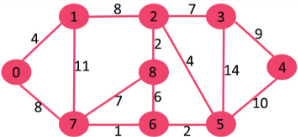The set mstSet is initially empty and keys assigned to vertices are {0, INF, INF, INF, INF, INF, INF, INF} where INF indicates infinite. Now pick the vertex with minimum key value. The vertex 0 is picked, include it in mstSet. So mstSet becomes {0}. After including to mstSet, update key values of adjacent vertices. Adjacent vertices of 0 are 1 and 7. The key values of 1 and 7 are updated as 4 and 8. Following subgraph shows vertices and their key values, only the vertices with finite key values are shown. The vertices included in MST are shown in green color.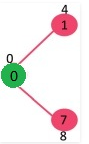Pick the vertex with minimum key value and not already included in MST (not in mstSET). The vertex 1 is picked and added to mstSet. So mstSet now becomes {0, 1}. Update the key values of adjacent vertices of 1. The key value of vertex 2 becomes 8.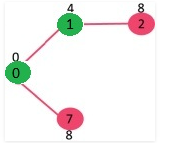Pick the vertex with minimum key value and not already included in MST (not in mstSET). We can either pick vertex 7 or vertex 2, let vertex 7 is picked. So mstSet now becomes {0, 1, 7}. Update the key values of adjacent vertices of 7. The key value of vertex 6 and 8 becomes finite (7 and 1 respectively).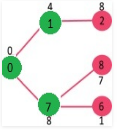Pick the vertex with minimum key value and not already included in MST (not in mstSET). Vertex 6 is picked. So mstSet now becomes {0, 1, 7, 6}. Update the key values of adjacent vertices of 6. The key value of vertex 5 and 8 are updated.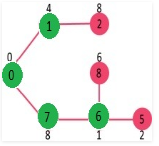We repeat the above steps until mstSet includes all vertices of given graph. Finally, we get the following graph.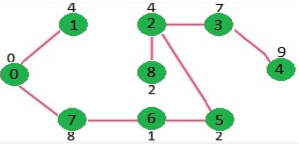How to implement the above algorithm?

We use a boolean array mstSet[] to represent the set of vertices included in MST. If a value mstSet[v] is true, then vertex v is included in MST, otherwise not. Array key[] is used to store key values of all vertices. Another array parent[] to store indexes of parent nodes in MST. The parent array is the output array which is used to show the constructed MST.

// A C / C++ program for Prim's Minimum Spanning Tree (MST) algorithm.
// The program is for adjacency matrix representation of the graph

#include <stdio.h>
#include <limits.h>

// Number of vertices in the graph
#define V 5

// A utility function to find the vertex with minimum key value, from
// the set of vertices not yet included in MST
int minKey(int key[], bool mstSet[])
{
// Initialize min value
int min = INT_MAX, min_index;

for (int v = 0; v < V; v++)
if (mstSet[v] == false && key[v] < min)
min = key[v], min_index = v;

return min_index;
}

// A utility function to print the constructed MST stored in parent[]
int printMST(int parent[], int n, int graph[V][V])
{
printf("Edge   Weight ");
for (int i = 1; i < V; i++)
printf("%d - %d    %d ", parent[i], i, graph[i][parent[i]]);
}

// Function to construct and print MST for a graph represented using adjacency
// matrix representation
void primMST(int graph[V][V])
{
int parent[V]; // Array to store constructed MST
int key[V];   // Key values used to pick minimum weight edge in cut
bool mstSet[V];  // To represent set of vertices not yet included in MST

// Initialize all keys as INFINITE
for (int i = 0; i < V; i++)
key[i] = INT_MAX, mstSet[i] = false;

// Always include first 1st vertex in MST.
key = 0;     // Make key 0 so that this vertex is picked as first vertex
parent = -1; // First node is always root of MST

// The MST will have V vertices
for (int count = 0; count < V-1; count++)
{
// Pick the minimum key vertex from the set of vertices
// not yet included in MST
int u = minKey(key, mstSet);

// Add the picked vertex to the MST Set
mstSet[u] = true;

// Update key value and parent index of the adjacent vertices of
// the picked vertex. Consider only those vertices which are not yet
// included in MST
for (int v = 0; v < V; v++)

// graph[u][v] is non zero only for adjacent vertices of m
// mstSet[v] is false for vertices not yet included in MST
// Update the key only if graph[u][v] is smaller than key[v]
if (graph[u][v] && mstSet[v] == false && graph[u][v] <  key[v])
parent[v]  = u, key[v] = graph[u][v];
}

// print the constructed MST
printMST(parent, V, graph);
}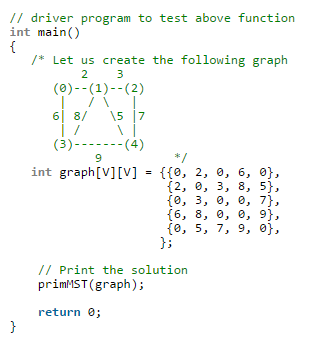Output:
Edge   Weight
0 - 1      2
1 - 2      3
0 - 3      6
1 - 4      5

Time Complexity of the above program is O(V^2). If the input  graph is represented using adjacency list, then the time complexity of Prim’s algorithm can be reduced to O(E log V) with the help of binary heap.

## Greedy Algorithms | (Dijkstra’s shortest path algorithm)

Given a graph and a source vertex in graph, find shortest paths from source to all vertices in the given graph.

Dijkstra’s algorithm is very similar to  prim`s algorithm for minimum apanning tree. Like Prim’s MST, we generate a SPT (shortest path tree) with given source as root. We maintain two sets, one set contains vertices included in shortest path tree, other set includes vertices not yet included in shortest path tree. At every step of the algorithm, we find a vertex which is in the other set (set of not yet included) and has minimum distance from source.

Below are the detailed steps used in Dijkstra’s algorithm to find the shortest path from a single source vertex to all other vertices in the given graph.
Algorithm
1) Create a set sptSet (shortest path tree set) that keeps track of vertices included in shortest path tree, i.e., whose minimum distance from source is calculated and finalized. Initially, this set is empty.
2) Assign a distance value to all vertices in the input graph. Initialize all distance values as INFINITE. Assign distance value as 0 for the source vertex so that it is picked first.
3) While sptSet doesn’t include all vertices
….a) Pick a vertex u which is not there in sptSetand has minimum distance value.
….b) Include u to sptSet.
….c) Update distance value of all adjacent vertices of u. To update the distance values, iterate through all adjacent vertices. For every adjacent vertex v, if sum of distance value of u (from source) and weight of edge u-v, is less than the distance value of v, then update the distance value of v.

Let us understand with the following example: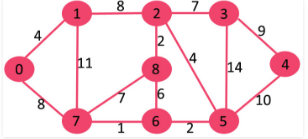The set sptSetis initially empty and distances assigned to vertices are {0, INF, INF, INF, INF, INF, INF, INF} where INF indicates infinite. Now pick the vertex with minimum distance value. The vertex 0 is picked, include it in sptSet. So sptSet becomes {0}. After including 0 to sptSet, update distance values of its adjacent vertices. Adjacent vertices of 0 are 1 and 7. The distance values of 1 and 7 are updated as 4 and 8. Following subgraph shows vertices and their distance values, only the vertices with finite distance values are shown. The vertices included in SPT are shown in green color.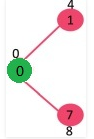Pick the vertex with minimum distance value and not already included in SPT (not in sptSET). The vertex 1 is picked and added to sptSet. So sptSet now becomes {0, 1}. Update the distance values of adjacent vertices of 1. The distance value of vertex 2 becomes 12.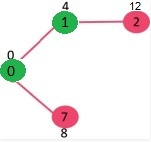Pick the vertex with minimum distance value and not already included in SPT (not in sptSET). Vertex 7 is picked. So sptSet now becomes {0, 1, 7}. Update the distance values of adjacent vertices of 7. The distance value of vertex 6 and 8 becomes finite (15 and 9 respectively).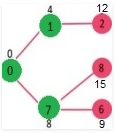Pick the vertex with minimum distance value and not already included in SPT (not in sptSET). Vertex 6 is picked. So sptSet now becomes {0, 1, 7, 6}. Update the distance values of adjacent vertices of 6. The distance value of vertex 5 and 8 are updated.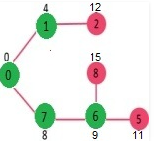We repeat the above steps until sptSet doesn’t include all vertices of given graph. Finally, we get the following Shortest Path Tree (SPT).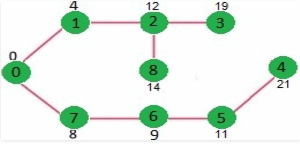How to implement the above algorithm?

We use a boolean array sptSet[] to represent the set of vertices included in SPT. If a value sptSet[v] is true, then vertex v is included in SPT, otherwise not. Array dist[] is used to store shortest distance values of all vertices.

// A C / C++ program for Dijkstra's single source shortest path algorithm.
// The program is for adjacency matrix representation of the graph

#include <stdio.h>
#include <limits.h>

// Number of vertices in the graph
#define V 9

// A utility function to find the vertex with minimum distance value, from
// the set of vertices not yet included in shortest path tree
int minDistance(int dist[], bool sptSet[])
{
// Initialize min value
int min = INT_MAX, min_index;

for (int v = 0; v < V; v++)
if (sptSet[v] == false && dist[v] <= min)
min = dist[v], min_index = v;

return min_index;
}

// A utility function to print the constructed distance array
int printSolution(int dist[], int n)
{
printf("Vertex   Distance from Source ");
for (int i = 0; i < V; i++)
printf("%d %d ", i, dist[i]);
}

// Funtion that implements Dijkstra's single source shortest path algorithm
// for a graph represented using adjacency matrix representation
void dijkstra(int graph[V][V], int src)
{
int dist[V];     // The output array.  dist[i] will hold the shortest
// distance from src to i

bool sptSet[V]; // sptSet[i] will true if vertex i is included in shortest
// path tree or shortest distance from src to i is finalized

// Initialize all distances as INFINITE and stpSet[] as false
for (int i = 0; i < V; i++)
dist[i] = INT_MAX, sptSet[i] = false;

// Distance of source vertex from itself is always 0
dist[src] = 0;

// Find shortest path for all vertices
for (int count = 0; count < V-1; count++)
{
// Pick the minimum distance vertex from the set of vertices not
// yet processed. u is always equal to src in first iteration.
int u = minDistance(dist, sptSet);

// Mark the picked vertex as processed
sptSet[u] = true;

// Update dist value of the adjacent vertices of the picked vertex.
for (int v = 0; v < V; v++)

// Update dist[v] only if is not in sptSet, there is an edge from
// u to v, and total weight of path from src to  v through u is
// smaller than current value of dist[v]
if (!sptSet[v] && graph[u][v] && dist[u] != INT_MAX
&& dist[u]+graph[u][v] < dist[v])
dist[v] = dist[u] + graph[u][v];
}

// print the constructed distance array
printSolution(dist, V);
}

// driver program to test above function
int main()
{
/* Let us create the example graph discussed above */
int graph[V][V] = {{0, 4, 0, 0, 0, 0, 0, 8, 0},
{4, 0, 8, 0, 0, 0, 0, 11, 0},
{0, 8, 0, 7, 0, 4, 0, 0, 2},
{0, 0, 7, 0, 9, 14, 0, 0, 0},
{0, 0, 0, 9, 0, 10, 0, 0, 0},
{0, 0, 4, 14, 10, 0, 2, 0, 0},
{0, 0, 0, 0, 0, 2, 0, 1, 6},
{8, 11, 0, 0, 0, 0, 1, 0, 7},
{0, 0, 2, 0, 0, 0, 6, 7, 0}
};

dijkstra(graph, 0);

return 0;
}

Output:
Vertex   Distance from Source
0                0
1                4
2                12
3                19
4                21
5                11
6                9
7                8
8                14

Notes:
1) The code calculates shortest distance, but doesn’t calculate the path information. We can create a parent array, update the parent array when distance is updated (like prim`s implementation) and use it show the shortest path from source to different vertices.

2) The code is for undirected graph, same dijkstra function can be used for directed graphs also.

3) The code finds shortest distances from source to all vertices. If we are interested only in shortest distance from source to a single target, we can break the for loop when the picked minimum distance vertex is equal to target (Step 3.a of algorithm).

4) Time Complexity of the implementation is O(V^2). If the input  graph is represented using adjacency list, it can be reduced to O(E log V) with the help of binary heap. Dijkstra`s Algorithm for Adjacency List Representation for more details.

5) Dijkstra’s algorithm doesn’t work for graphs with negative weight edges. For graphs with negative weight edges, Bellman-Ford algorithm can be used, we will soon be discussing it as a separate post.

Offer running on EduRev: Apply code STAYHOME200 to get INR 200 off on our premium plan EduRev Infinity!

## GATE Computer Science Engineering(CSE) 2022 Mock Test Series

131 docs|167 tests

,

,

,

,

,

,

,

,

,

,

,

,

,

,

,

,

,

,

,

,

,

;